Скачать презентацию Lecture1

lecture1.ppt

• Размер: 207 Кб
• Количество слайдов: 29

## Описание презентации Презентация Lecture1 по слайдам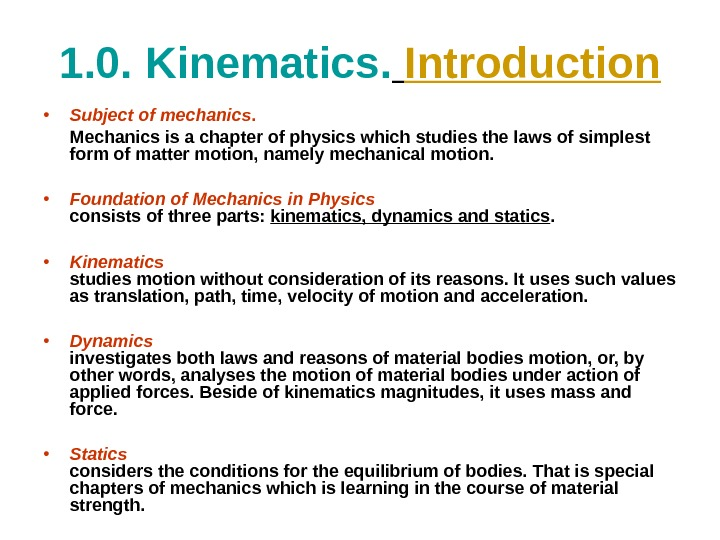1. 0. Kinematics. Introduction • Subject of mechanics. Mechanics is a chapter of physics which studies the laws of simplest form of matter motion, namely mechanical motion. • Foundation of Mechanics in Physics consists of three parts: kinematics, dynamics and statics. • Kinematics studies motion without consideration of its reasons. It uses such values as translation, path, time, velocity of motion and acceleration. • Dynamics investigates both laws and reasons of material bodies motion, or, by other words, analyses the motion of material bodies under action of applied forces. Beside of kinematics magnitudes, it uses mass and force. • Statics considers the conditions for the equilibrium of bodies. That is special chapters of mechanics which is learning in the course of material strength.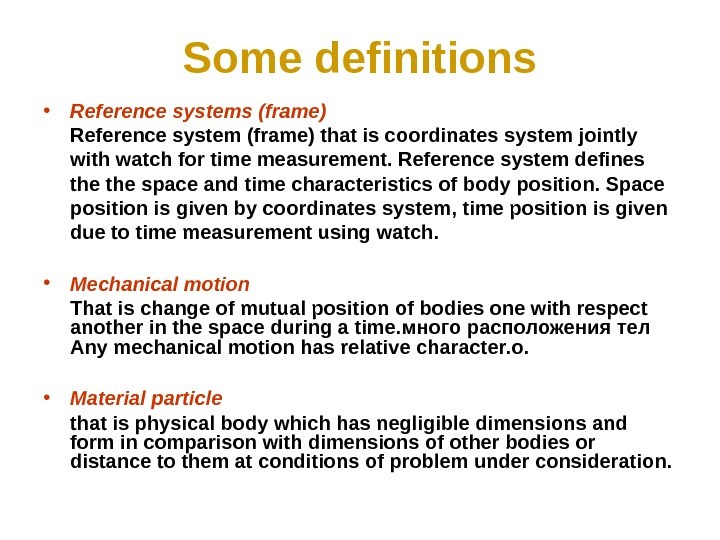Some definitions • Reference systems (frame) Reference system (frame) that is coordinates system jointly with watch for time measurement. Reference system defines the space and time characteristics of body position. Space position is given by coordinates system , time position is given due to time measurement using watch. • Mechanical motion That is change of mutual position of bodies one with respect another in the space during a time. много расположения тел Any mechanical motion has relative character. о. • Material particle that is physical body which has negligible dimensions and form in comparison with dimensions of other bodies or distance to them at conditions of problem under consideration.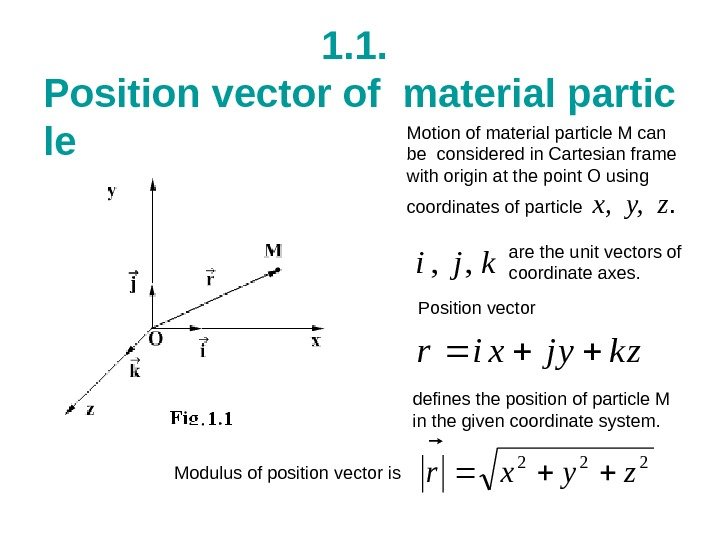1. 1. Position vector of material partic le Motion of material particle M can be considered in Cartesian frame with origin at the point O using coordinates of particle x, y, z. are the unit vectors of coordinate axes. Position vector defines the position of particle M in the given coordinate system. kji , , zkyjxir 222 zyxr Modulus of position vector is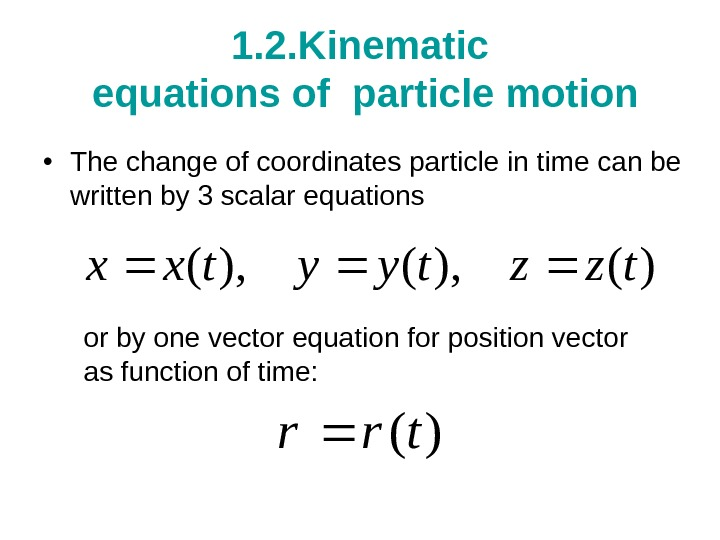1. 2. Kinematic equations of particle motion • The change of coordinates particle in time can be written by 3 scalar equations or by one vector equation for position vector as function of time: )(), (tzztyytxx )(trr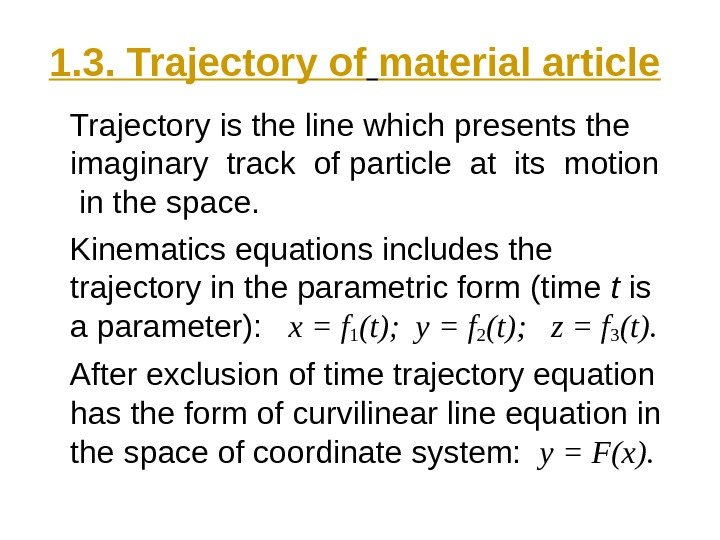1. 3. Trajectory of material article Trajectory is the line which presents the imaginary track of particle at its motion in the space. Kinematics equations includes the trajectory in the parametric form (time t is a parameter): x = f 1 (t); y = f 2 (t); z = f 3 (t). After exclusion of time trajectory equation has the form of curvilinear line equation in the space of coordinate system: y = F(x).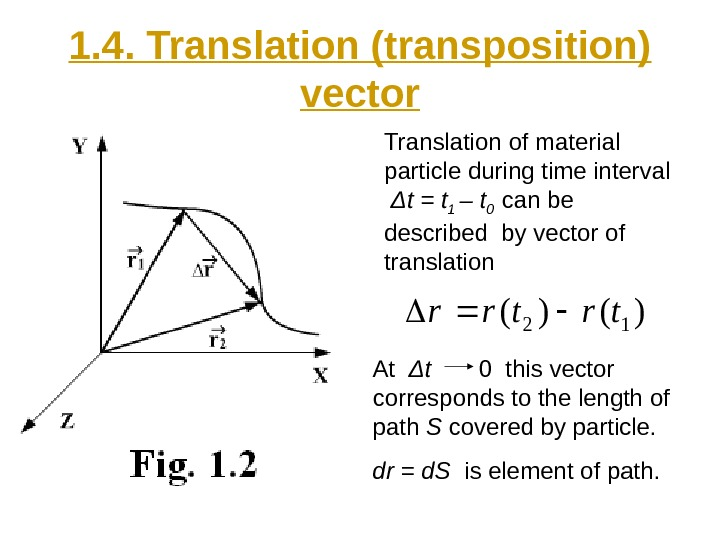1. 4. Translation (transposition) vector Translation of material particle during time interval Δ t = t 1 – t 0 can be described by vector of translation At Δ t 0 this vector corresponds to the length of path S covered by particle. dr = d. S is element of path. )()(12trtrr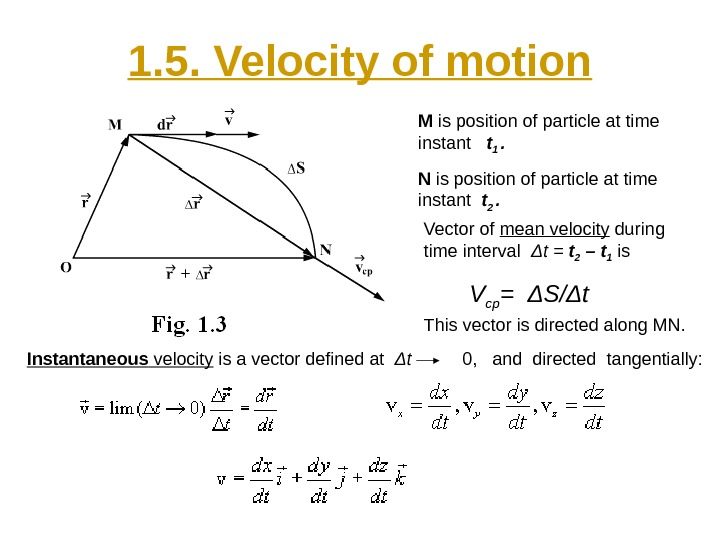1. 5. Velocity of motion M is position of particle at time instant t 1 . N is position of particle at time instant t 2 . Vector of mean velocity during time interval Δ t = t 2 – t 1 is V ср = Δ S/ Δ t This vector is directed along MN. Instantaneous velocity is a vector defined at Δ t 0, and directed tangentially: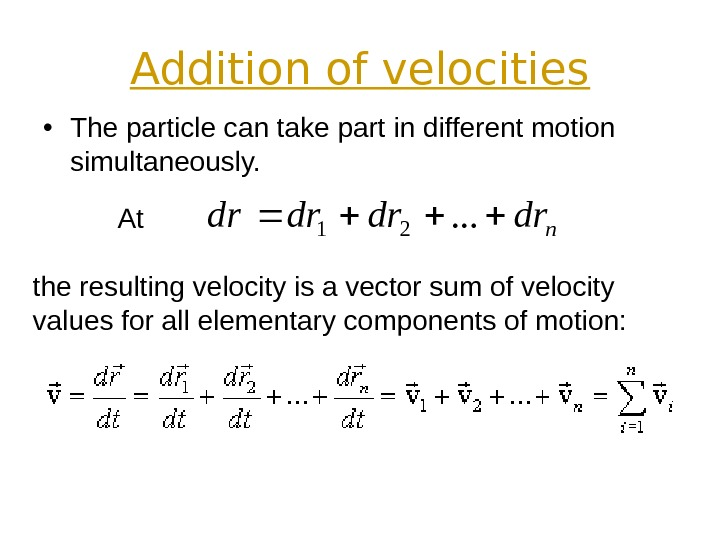Addition of velocities • The particle can take part in different motion simultaneously. At the resulting velocity is a vector sum of velocity values for all elementary components of motion: nrdrd . . .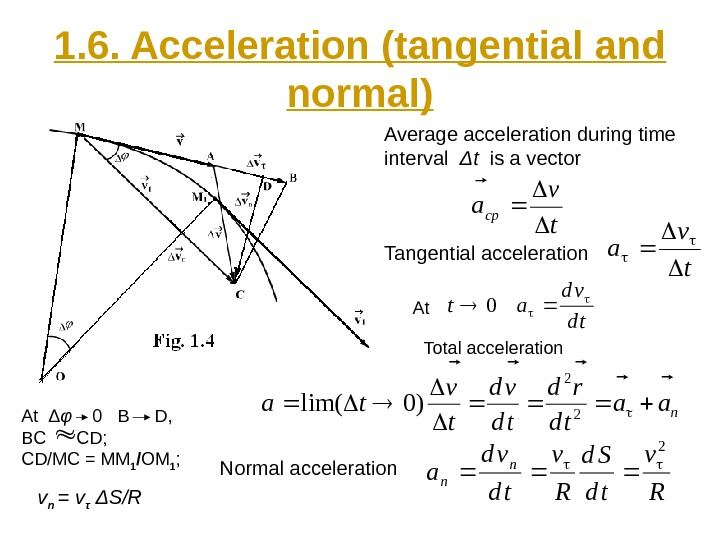1. 6. Acceleration (tangential and normal) Average acceleration during time interval Δ t is a vector Tangential acceleration tv a Total acceleration td vd at 0At naa td rd td vd t v ta 2 2 )0lim( At Δ φ 0 B D, BC CD; CD/MC = MM 1 / OM 1 ; v n = v τ Δ S/R Normal acceleration R v td Sd R v td vd a n n 2 t v acp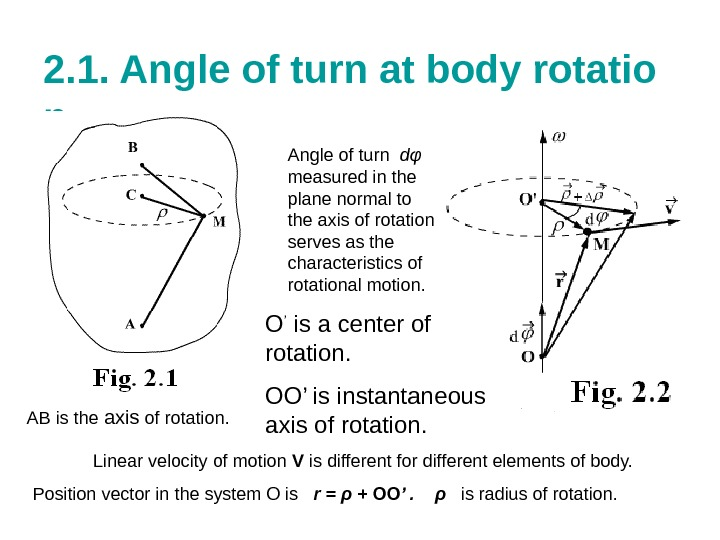2. 1. Angle of turn at body rotatio n AB is the axis of rotation. Angle of turn d φ measured in the plane normal to the axis of rotation serves as the characteristics of rotational motion. Ο ’ is a center of rotation. O Ο’ is instantaneous axis of rotation. Linear velocity of motion V is different for different elements of body. Position vector in the system O is r = ρ + ΟΟ ’. ρ is radius of rotation.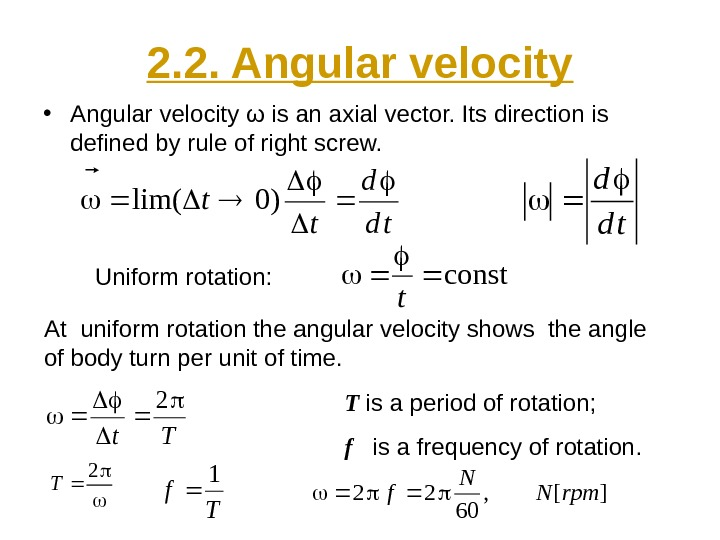2. 2. Angular velocity • Angular velocity ω is an axial vector. Its direction is defined by rule of right screw. td d t t )0lim( Uniform rotation: const t td d At uniform rotation the angular velocity shows the angle of body turn per unit of time. Tt 2 2 T T f 1 ][, 60 22rpm. N N f T is a period of rotation; f is a frequency of rotation.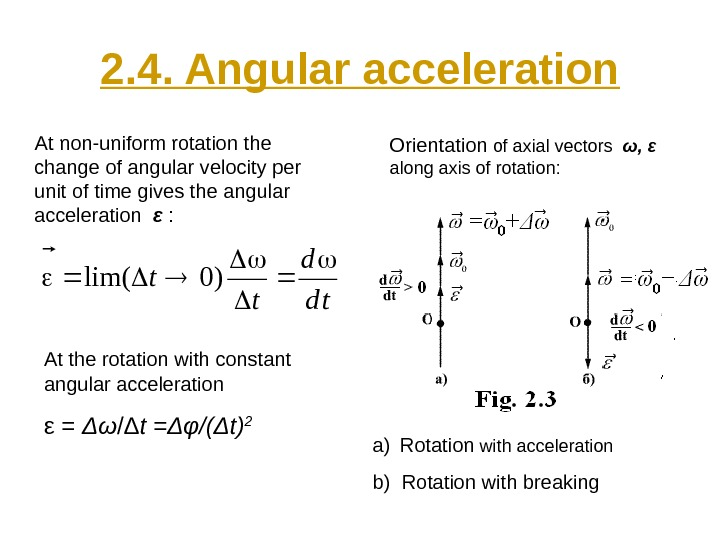2. 4. Angular acceleration At non-uniform rotation the change of angular velocity per unit of time gives the angular acceleration ε : Orientation of axial vectors ω, ε along axis of rotation: td d t t )0lim( a) Rotation with acceleration b) Rotation with breaking. At the rotation with constant angular acceleration ε = Δω /Δ t = Δφ/(Δ t)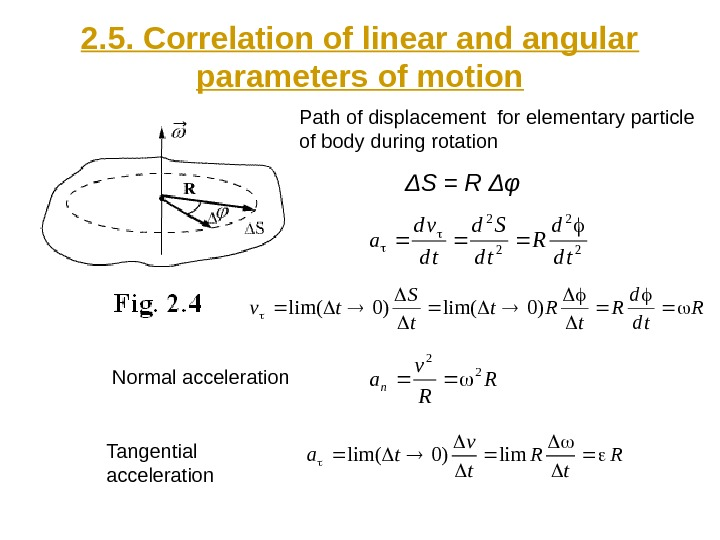2. 5. Correlation of linear and angular parameters of motion Path of displacement for elementary particle of body during rotation Δ S = R ΔφR td d R t Rt t S tv )0lim( R R v an 2 2 R t v ta lim)0lim( Normal acceleration Tangential acceleration 2 2 td d R td Sd td vd a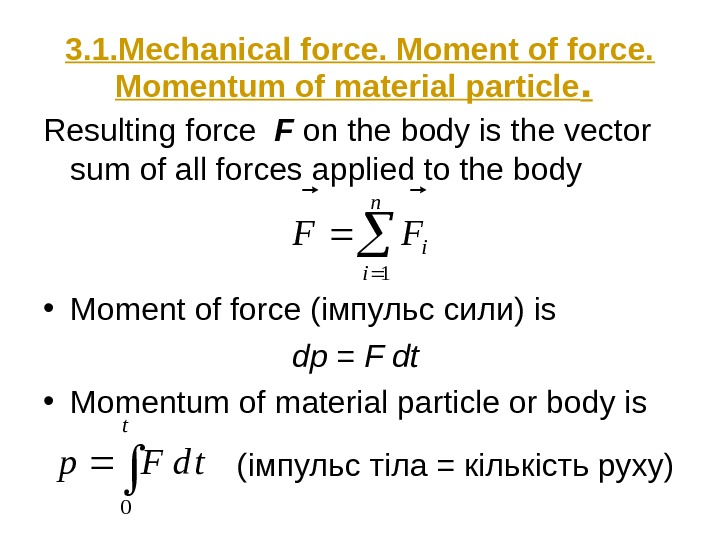3. 1. Mechanical force. Moment of force. Momentum of material particle. Resulting force F on the body is the vector sum of all forces applied to the body • Moment of force ( імпульс сили) is dp = F dt • Momentum of material particle or body is ttd. Fp 0 n i i. FF 1 (імпульс тіла = кількість руху)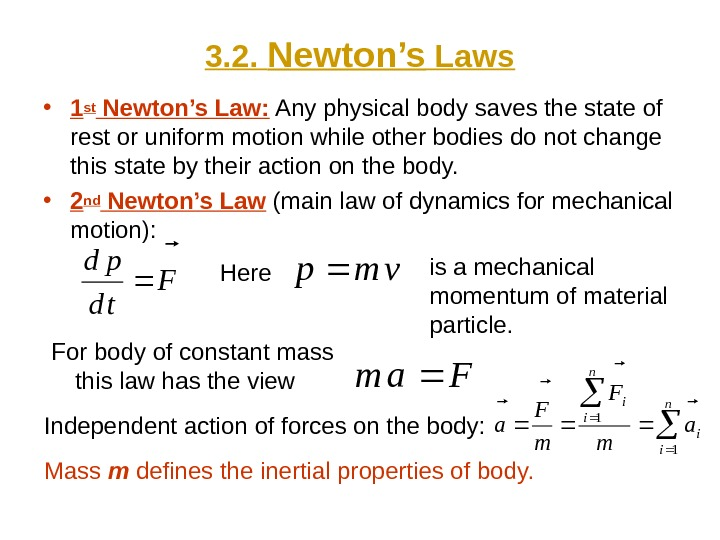3. 2. Newton’s Laws • 1st Newton’s Law: Any physical body saves the state of rest or uniform motion while other bodies do not change this state by their action on the body. • 2 nd Newton’s Law (main law of dynamics for mechanical motion): F td pd Herevmp is a mechanical momentum of material particle. For body of constant mass this law has the view Fam Independent action of forces on the body: n i i a m F a 1 1 Mass m defines the inertial properties of body.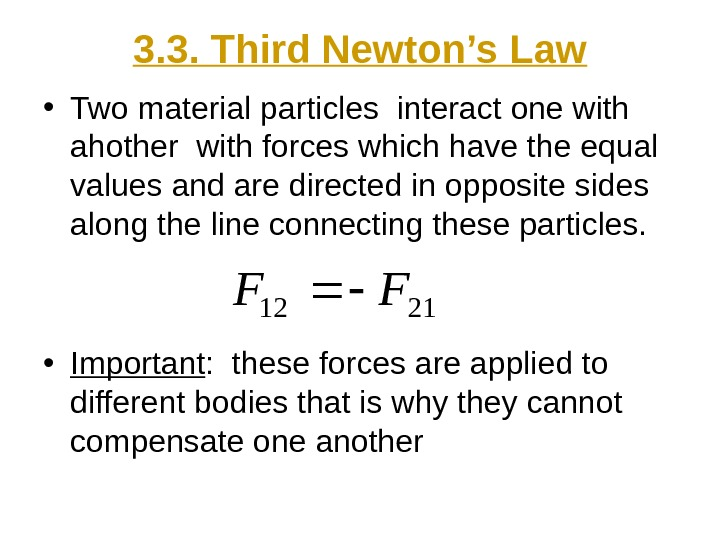3. 3. Third Newton’s Law • Two material particles interact one with ahother with forces which have the equal values and are directed in opposite sides along the line connecting these particles. • Important : these forces are applied to different bodies that is why they cannot compensate one another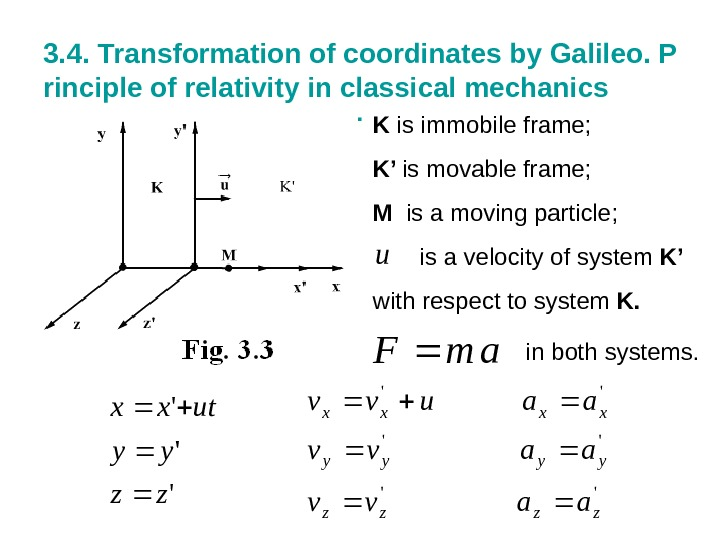3. 4. Transformation of coordinates by Galileo. P rinciple of relativity in classical mechanics. K is immobile frame; K’ is movable frame; M is a moving particle; is a velocity of system K’ with respect to system K. u ‘ ‘ ‘ zz yy utxx » » » zzzz yyyy xxxx aavv aauvv am. F in both systems.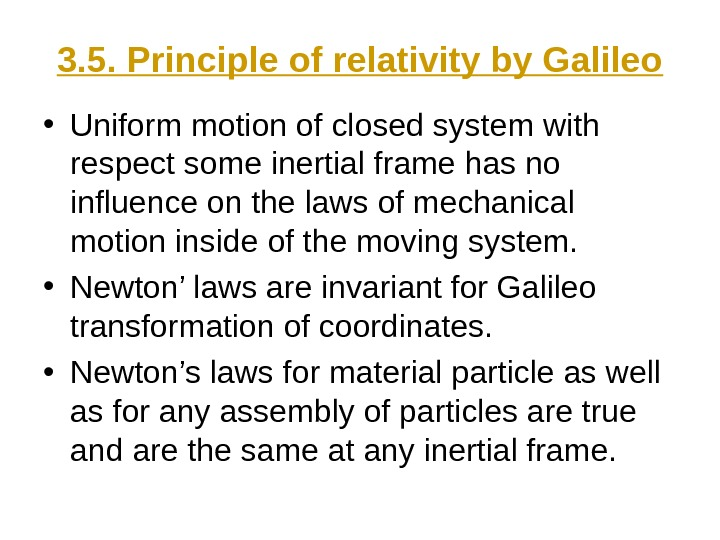3. 5. Principle of relativity by Galileo • Uniform motion of closed system with respect some inertial frame has no influence on the laws of mechanical motion inside of the moving system. • Newton’ laws are invariant for Galileo transformation of coordinates. • Newton’s laws for material particle as well as for any assembly of particles are true and are the same at any inertial frame.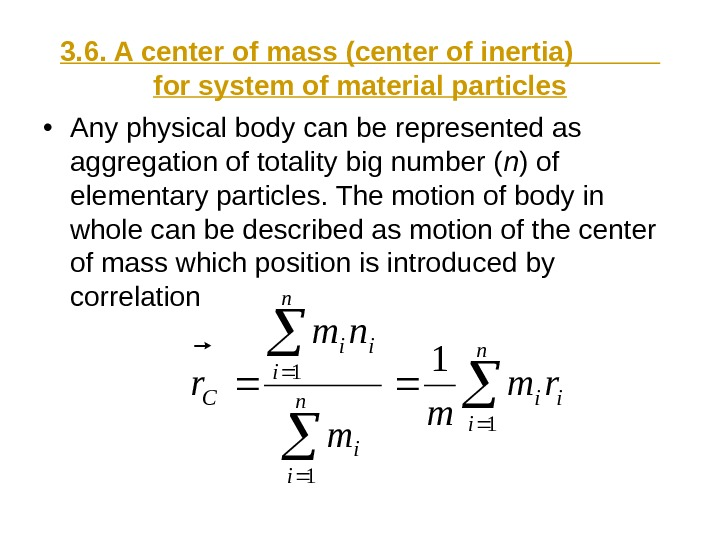3. 6. A center of mass (center of inertia) for system of material particles • Any physical body can be represented as aggregation of totality big number ( n ) of elementary particles. The motion of body in whole can be described as motion of the center of mass which position is introduced by correlation i n i ii Crm m m nm r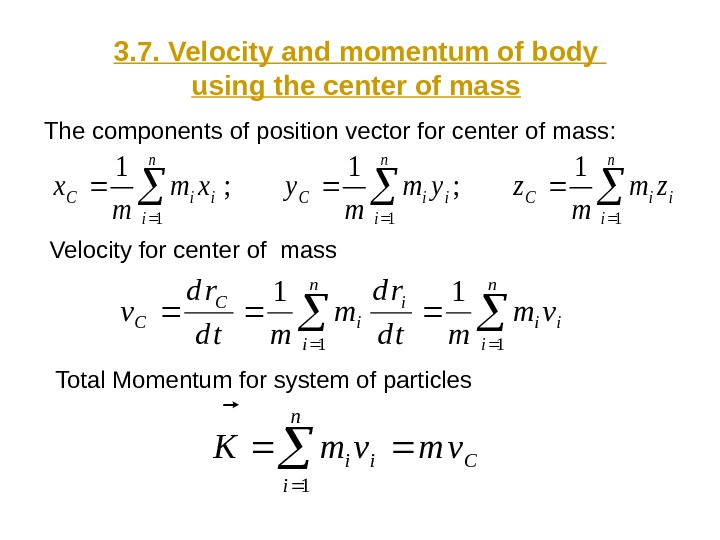3. 7. Velocity and momentum of body using the center of mass The components of position vector for center of mass: i n i i. Ci n i i. Czm m zym m yxm m x 111 1 ; 1 n i ii i n i i C Cvm mtd rd v 11 11 Velocity for center of mass Total Momentum for system of particles C n i iivmvm. K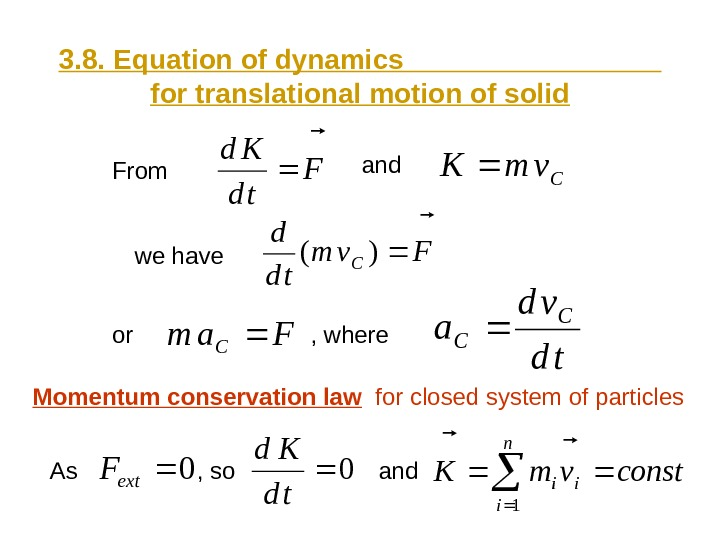3. 8. Equation of dynamics for translational motion of solid. F td Kd Cvm. K Fvm td d C )( Fam C td vd a C CFrom and we have or , where Momentum conservation law for closed system of particles 0ext. F 0 td Kd constvm. K n i ii 1 and, so. As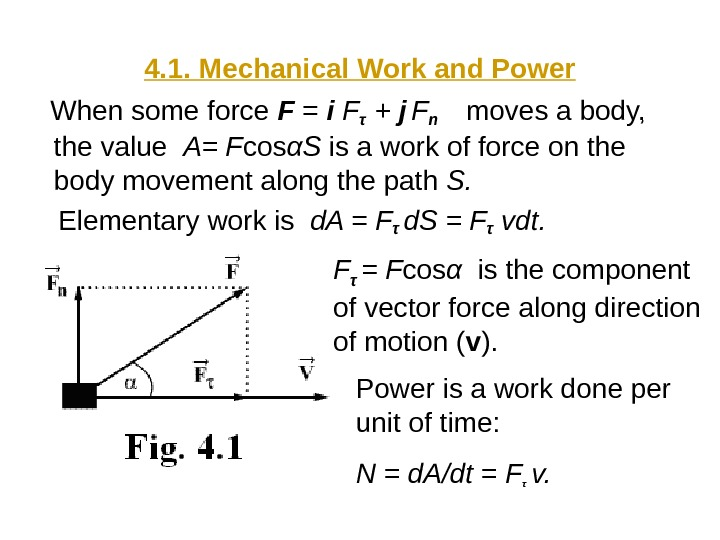4. 1. Mechanical Work and Power When some force F = i Fτ + j Fn moves a body, the value A= F cos α S is a work of force on the body movement along the path S. Elementary work is d. A = F τ d. S = Fτ vdt. F τ = F cos α is the component of vector force along direction of motion ( v ). Power is a work done per unit of time: N = d. A/dt = F τ v.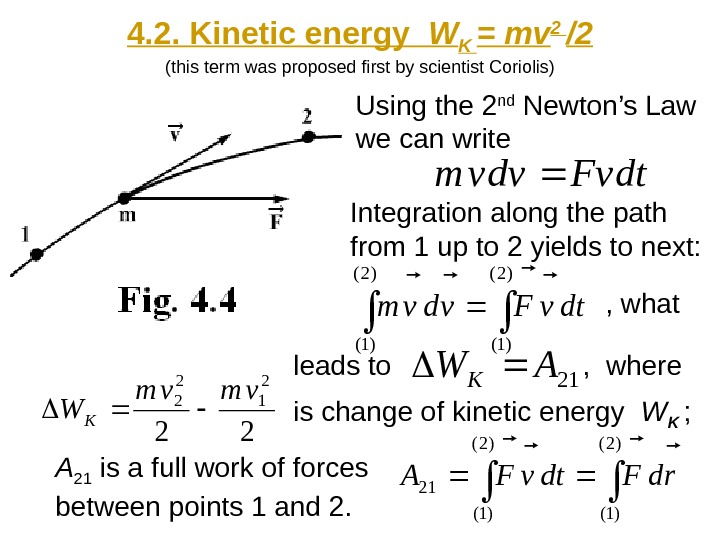4. 2. Kinetic energy W K = mv 2 /2 (this term was proposed first by scientist Coriolis) Using the 2 nd Newton’s Law we can write Integration along the path from 1 up to 2 yields to next: dtv. Fvdvm )2( )1( dtv. Fvdvm leads to 21AWK , where 22 2 1 2 2vmvm WK is change of kinetic energy W K ; )2( )1( 21rd. Fdtv. FA A 21 is a full work of forces between points 1 and 2. , what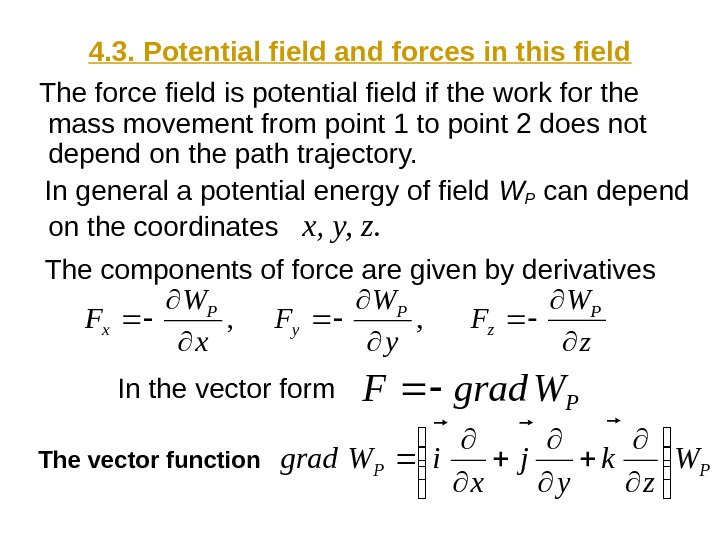4. 3. Potential field and forces in this field The force field is potential field if the work for the mass movement from point 1 to point 2 does not depend on the path trajectory. In general a potential energy of field WP can depend on the coordinates x, y, z. The components of force are given by derivatives , x W F P x , y W F P y z W F P z PWgrad. F PPW z k y j x i. Wgrad In the vector form The vector function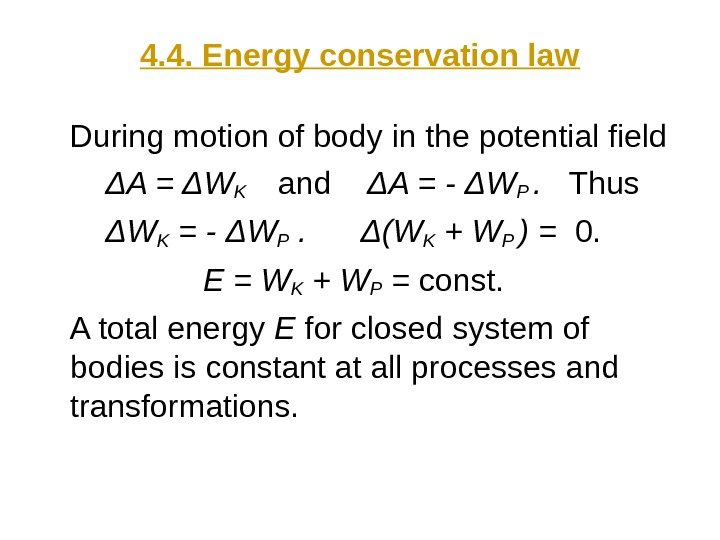4. 4. Energy conservation law During motion of body in the potential field ΔΑ = Δ WK and Δ A = — Δ WP . Thus Δ W K = — Δ WP . Δ( WK + WP ) = 0. E = W K + WP = const. A total energy E for closed system of bodies is constant at all processes and transformations.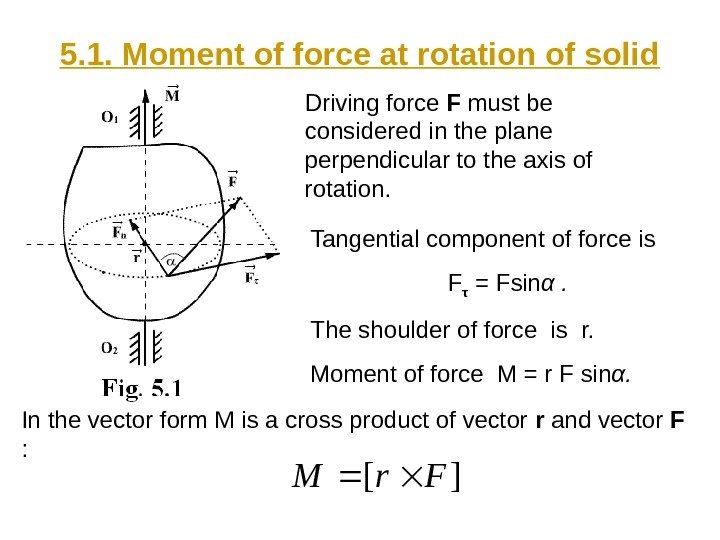5. 1. Moment of force at rotation of solid Driving force F must be considered in the plane perpendicular to the axis of rotation. Tangential component of force is F τ = Fsin α. The shoulder of force is r. Moment of force M = r F sin α. ][Fr. M In the vector form M is a cross product of vector r and vector F :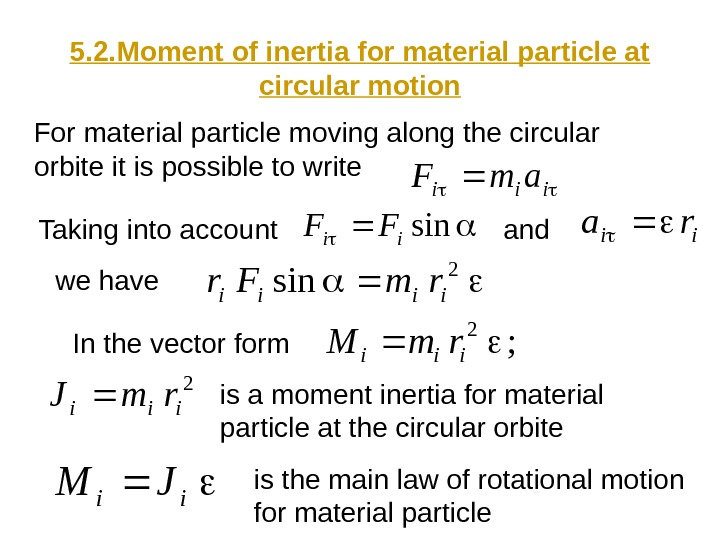5. 2. Moment of inertia for material particle at circular motion For material particle moving along the circular orbite it is possible to write iiiam. F Taking into account sinii. FF ii ra and we have 2 siniiiirm. Fr In the vector form ; 2 iiirm. M 2 iiirm. J is a moment inertia for material particle at the circular orbite ii JM is the main law of rotational motion for material particle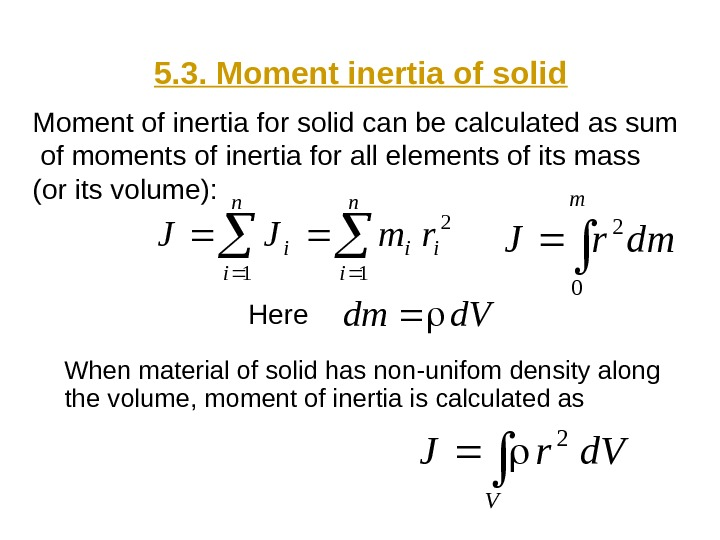5. 3. Moment inertia of solid When material of solid has non-unifom density along the volume, moment of inertia is calculated as n i ii n i irm. JJ 1 2 1 dmr. J m 0 2Moment of inertia for solid can be calculated as sum of moments of inertia for all elements of its mass (or its volume): V d. Vr. J 2 Here d. Vdm